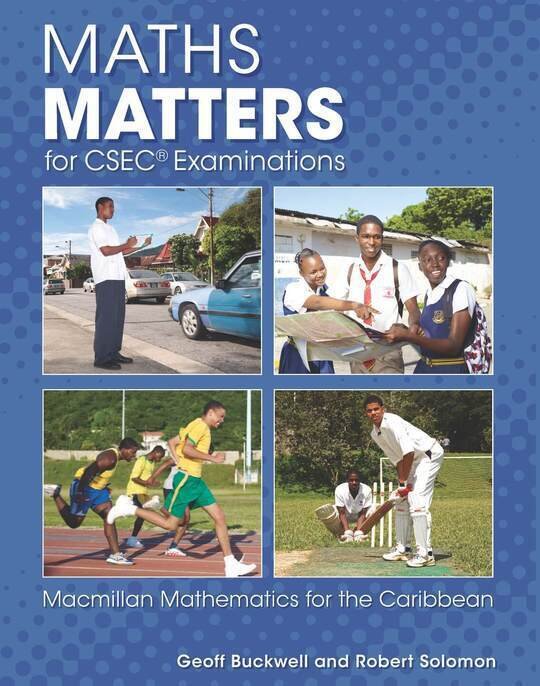Macmillan EducationGeoff Buckwell
Test Preparation
Maths Matters for CSEC® Examinations
US\$ 26.31
The publisher has enabled DRM protection, which means that you need to use the BookFusion iOS, Android or Web app to read this eBook. This eBook cannot be used outside of the BookFusion platform.
Description
Contents
Reviews

Language
English
ISBN
9780230464957
Cover
Title
Contents
Introduction
Scope and sequence
1 Numbers theory
1.1 Classifiying numbers
1.2 Operations, identity and inverse
1.3 Closure
1.4 Distributive law and associative law in number problems
1.5 Other bases (revision)
2 Rounding and errors
2.1 Significant figures, decimal places and standard form
2.2 Errors in measurement
2.3 Rounding effects when substituting in formulae
3 Consumer arithmetic
3.1 Income
3.2 Taxes
3.3 Utilities
3.4 Currency
3.5 Paying by instalments
3.6 Interest
4 Sets and sequences
4.1 Definitions
4.2 Sequences
4.3 Finding the rule of a sequence
5 Algebra: expressions
5.1 Algebraic operations
5.2 Indices
5.3 Binary operations
5.4 Algebraic fractions
6 Algebra: equations
6.1 Linear equations
6.2 Simultaneous equations
6.3 Inequalities
6.4 Changing the subject
7 Coordinate geometry of the straight line
7.1 Straight-line graphs
7.3 Gradients and intercepts of straight lines
7.4 Finding the equation of a straight line
7.5 Parallel and perpendicular lines
7.6 Distance between points
7.7 Midpoints
7.8 Graphical solution of simultaneous equations
7.9 Inequalities in two dimensions
8.1 Expanding a bracket (revision)
8.2 Expanding pairs of brackets
8.3 Identities
8.4 Factorising two terms (revision)
8.6 The general quadratic ax[sup(2)] + bx + c
9 Ratio and proportion
9.1 Ratio
9.2 Rate
9.3 Direct proportion
9.4 Inverse proportion
9.5 Proportion to a power
10 Geometry 1
10.1 Lines, angles, polygons and solids
10.2 Basic drawing and constructions
10.3 Symmetry
11 Geometry problems
11.1 Lines, angles and polygons
11.2 Congruent triangles
11.3 Similar figures
12 Transformations
12.1 Translations using vectors
12.2 Transformations (revision)
12.3 Combining transformations
12.4 Glide reflections
13 Surface area and volume
13.1 Revision of units
13.2 Length and area
13.3 Area of regular shapes (revision)
13.4 Surface area
13.5 Volume
13.6 The cylinder
13.7 Pyramids and cones
13.8 The sphere
13.9 Compound shapes
14 Trigonometry
14.1 Pythagoras' theorem
14.2 The trigonometric ratios
14.3 Bearings
14.4 The sine rule
14.5 Using the sine rule to find angles
14.6 The cosine rule
14.7 Using the cosine rule to find angles
14.8 Solving triangles
15 Circle theorems
15.1 Definitions
15.2 Angle at the centre of a circle
15.3 Angle in a semicircle
15.4 Angles in the same segment
15.6 Mixed examples
15.7 Tangents
15.8 Alternate segment theorem
15.9 Midpoint of a chord
16 Functions
16.1 Domain and range
16.2 Composite functions
16.3 Formulae for composite functions
16.4 Inverse functions
17 Statistics
17.1 Data and frequency tables
17.2 Statistical diagrams
17.3 Measures of central tendency
17.4 Range and interquartile range
17.5 Median and quartiles from a frequency table
17.6 Cumulative frequency
17.7 Probability
18 Vectors
18.1 The arithmetic of vectors and geometry
18.2 Position vectors
18.3 Magnitude of a vector, unit vectors
18.4 Midpoints
19 Matrix algebra
19.1 Arrays of numbers
19.3 Multiplication by a scalar
19.4 Matrix multiplication
19.5 The identity matrix
19.6 Inverse matrices
Optional special objectives
20.1 Completing the square
20.2 Greatest or least value and line of symmetry
20.3 Solving equations by completing the square
20.4 Line of symmetry and turning points by sketching
20.5 Simultaneous equations: one linear, one quadratic
21 Regions and linear programming
21.1 Inequalities revision
21.2 Revision of y = mx + c
21.3 Regions
21.4 Regions with sides not parallel to the axes
21.5 Situations which give rise to inequalities
21.6 Listing the possibilities
21.7 Linear programming
22 Further graphs
22.1 Graphs of powers of x
22.2 Distance–time graphs
22.3 Acceleration
22.4 Velocity–time graphs
23 Measurement, geometry and trigonometry
23.1 Area of a triangle
23.2 Area of a segment
23.3 Lines and planes
23.4 Lengths and angles in three dimensions
23.5 Lengths within solids
23.6 Angle between lines
24 Vectors and matrices
24.1 Equivalent (equal) and null vectors
24.2 Collinear points
24.3 Transformations using matrices
24.4 Enlargements
24.5 The unit square
24.6 Common matrices
24.7 Successive transformations using matrices
24.8 Simultaneous equations
Model examination papers
Glossary
A
B
C
D
E
F
G
H
I
K
L
M
O
P
Q
R
S
T
U
V
Index
A
B
C
D
E
F
G
H
I
K
L
M
N
O
P
Q
R
S
T
U
V
W
Y
Z
Back Cover
The book hasn't received reviews yet.